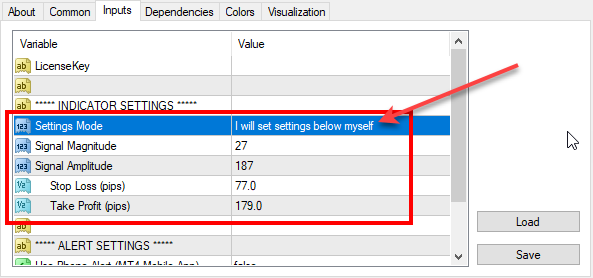# Re-optimized FxMagnetic indicator on EURUSD (2019-02-01)

We’ve reoptimized FxMagnetic indicator settings on EURUSD. Here are the latest parameters for all EURUSD timeframes that you should use for best MT4 indicator performance.To set parameters manually you need to switch the Settings Mode to: I will set settings below myself

Don’t have FxMagnetic indicator yet? You can buy FxMagnetic here.

## 1-minute chart:

Signal Magnitude = 20

Signal Amplitude = -180

Stop Loss (pips) = 35

Take Profit (pips) = 50

## 5-minute chart:

Signal Magnitude = 20

Signal Amplitude = -121

Stop Loss (pips) = 63

Take Profit (pips) = 113

## 15-minute chart:

Signal Magnitude = 92;

Signal Amplitude = -215;

Stop Loss (pips) = 64;

Take Profit (pips) = 105;

## 30-minute chart:

Signal Magnitude = 20;

Signal Amplitude = -137;

Stop Loss (pips) = 62;

Take Profit (pips) = 146;

## 1-hour chart:

Signal Magnitude = 20;

Signal Amplitude = -241;

Stop Loss (pips) = 74;

Take Profit (pips) = 91;

## 4-hour chart:

Signal Magnitude = 40;

Signal Amplitude = -140;

Stop Loss (pips) = 109;

Take Profit (pips) = 186;

## Daily chart:

Signal Magnitude = 98;

Signal Amplitude = -38;

Stop Loss (pips) = 300;

Take Profit (pips) = 482;

## Weekly chart:

Signal Magnitude = 20;

Ind0Param1 = -40;

Stop Loss (pips) = 360;

Take Profit (pips) = 1000;

## Monthly chart:

Signal Magnitude = 20;

Signal Amplitude = -80;

Stop Loss (pips) = 360;

Take Profit (pips) = 1000;

## FxMagnetic EURUSD v2.6 stats on 1st February, 2019

Below are the screenshots of FxMagnetic EURUSD stats from the last 100 signals. This was taken on 2019-02-01 on FxOpen Live ECN account. We re-optimized FxMagnetic EURUSD v2.6 on this broker so you’ll see best signals with them.

## New features in FxMagnetic EURUSD v2.6 indicator

The new FxMagnetic EURUSD v2.6 displays the date and time of the oldest signal on the chart. This is very useful because now you will have a clue how much time it took for the FxMagnetic to generate all previous 100 signals on the chart to produce the results.

The new FxMagnetic EURUSD also displays current signal direction and previous closed signal Profit/Loss figure in the same colors as the arrows on the chart. This helps you spot signal information easier.

1.Stefan Ferreia on February 1, 2019 at 3:09 pm
•FxMagnetic on February 1, 2019 at 3:16 pm